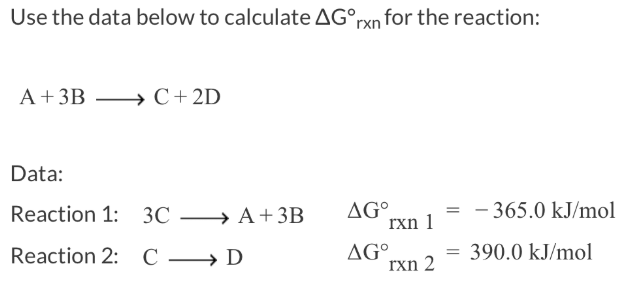# Problem: Use the data below to calculate ΔG°rxn for the reaction: A + 3B → C + 2D Data:Reaction 1: 3C → A + 3B ΔG°rxn1 = -365.0kJ/molReaction 2: C → D ΔG°rxn2 = 390 0 kJ/mol

###### FREE Expert Solution
81% (112 ratings)###### Problem Details

Use the data below to calculate ΔG°rxn for the reaction:

A + 3B → C + 2D

Data:
Reaction 1: 3C → A + 3B ΔG°rxn1 = -365.0kJ/mol
Reaction 2: C → D ΔG°rxn2 = 390 0 kJ/mol